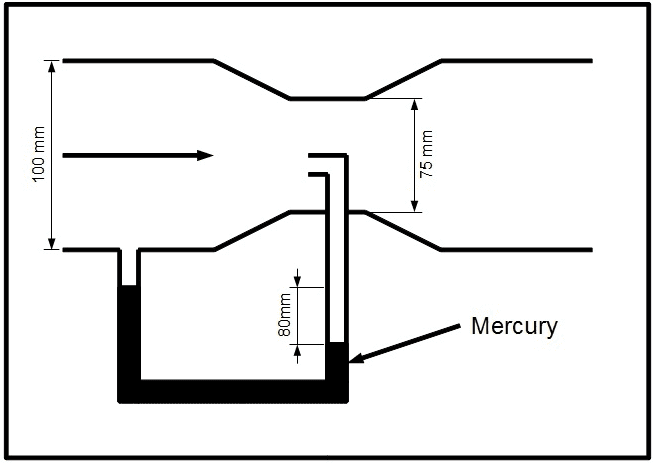# [Basic Hydraulics] The Pitot Tube/Manometers

• MarleyDH

## Homework Statement

Determine the flow rate, Q.

Ignore energy losses.

Given:

D1 = 100 mm
D2 = 75 mm
Fluid in the pipe is water.
Fluid in the metre is mercury.
S.G. of Mercury is ~13.6.
The difference in height of the mecury columns is 80mm.

Diagram is below.## Homework Equations

Bernoulli's Equation.

Equation of Continuity. (Q1 = Q2)

## The Attempt at a Solution

I approached this as a manometer question, but with that orifice in the flow I was unsure on how to proceed. I chose a datum as the lower of the two levels of mercury. The expressions I got were:

Px-x = Pressure due to the flow + Pressure due to the height of the water above the mercury + the pressure due to the mercury above the datum. This was for the left hand side.
Px-x = P1 + ρwgh + ρmgh', where h' = 80 mm and h is an unknown height which cancels out when the left side and right side are equated.

For the right hand side I said

Px-x = Pressure due to the flow + Pressure due to the height of the water.
Px-x = P2 + ρwg(h+h')

For this second equation I feel there is something missing, surely that opening in the flow causes an additional term to be added?